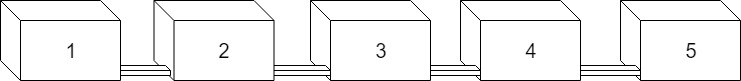# Simple Spatial Modeling – Part 1: Exercises

April 26, 2018
By

Want to share your content on R-bloggers? click here if you have a blog, or here if you don't.

This exercise is an extension of the last two previous exercises about numerical modeling. They can be found here and here. Those two previous exercises are representing how the model works in a lumped system. At this time, we will try to bring a space into our model. Make sure that you look at the latest exercise in numerical modeling so that we can recall the latest built model.

Instead of playing with parameter and loop calculations, we will develop our single water tank into a network. Assume that we add another two similar water tanks that are connected through pipes. It could represent the layers of soil or different areas across a catchment. Below is the illustration of the model. The output of this exercise is to understand how space can be illustrated by a model.Answers to these exercises are available here. If you obtained a different (correct) answer than those listed on the solutions page, please feel free to post your answer as a comment on that page.

Before starting the real exercises, try to load the data-set and plot it to see the data characteristic.

Exercise 1
Set the initial time as 0, with timestep = 1 and final time step = 100. Define the parameters of each water tank including k (parameter) and s (initial water level) and create an empty output `matrix`, this time, with four columns.

Exercise 2
Similar to before, try to run the loop through the number of time-steps.

Exercise 3
Plot the model output for each water tank; pay attention to the range of the result.

You can try to play with longer time-steps and varieties in the parameter to see if the model is realistic enough to predict the future.

What if the water tank arrangement has two-way interactions? In many situations, water flow direction varies over time, such as water flow in a soil profile under different conditions. The figure below is the schematic diagram of the next model.On the diagram above, each tank has a hole that connects to the adjacent bucket. If the water level in tank 1 is higher than tank 2, water will obviously flow from tank 1 into 2 until the water levels are equal and vice versa. So, water will flow in either direction. The rate of flow (q) depends on the difference between two tanks (H1-H2) and the size of the hole (k) or pipe that connect the two tanks, written mathematically as follows:
q = k x (H1-H2) x t

Assume that there is no hole on the outside of either bucket 1 or 5. So that k, q1, and q6 are zero.

Clear the previous values before starting the following exercises.
Exercise 4
Define initial conditions, including t, time-steps, final time-steps, and ncell as the number of water tanks available. Create an empty `matrix` of the water level (H) and the water flux output (q).

Exercise 5
Set the initial parameter for H and k.

Exercise 6
Try to use the `sequence` function to declare time-steps.

Exercise 7
Run the loop based on the parameters and the initial condition above.

Exercise 8
Plot the water level for each water tank. Pay attention to the output range.
Fancy to use a loop in water level plotting, huh?

Does the model meet your expectations?
Try to play around with the parameters and see how the model responds with those alterations.

R-bloggers.com offers daily e-mail updates about R news and tutorials about learning R and many other topics. Click here if you're looking to post or find an R/data-science job.
Want to share your content on R-bloggers? click here if you have a blog, or here if you don't.